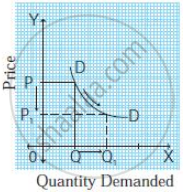# Identify and define the degrees of elasticity of demand from the following demand curve. - Economics

Short Note

Identify and define the degrees of elasticity of demand from the following demand curve.#### Solution

Unitary elastic demand: When the proportionate or percentage change in demand for a commodity is the same as the proportionate or percentage change in its price, it is called a unitary elastic demand. In this case, Ed = 1.

Concept: Types of Price Elasticity of Demand
Is there an error in this question or solution?

#### APPEARS IN

Balbharati Economics 12th Standard HSC Maharashtra State Board
Chapter 3.2 Elasticity of Demand
Exercise | Q 7. (1)(c) | Page 36